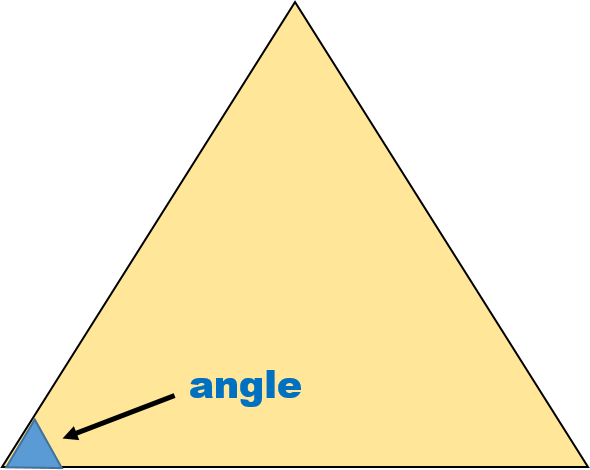1
visibility

Which of the following shapes has 5 angles?

• A• BThe correct answer is.

An angle measures the amount if turn. It is formed where two sides of a shape meet.

See example:We count the total number of angles.

There are 3 angles.

Solution:

Consider the shape below:We count the total number of angles.

There are 4 angles.

Consider the shape below:We count the total number of angles.

There are 5 angles.

So,has 5 angles.